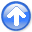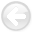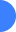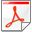Wave-equation tomography by beam focusingNext: Introduction Up: Reproducible Documents

# Wave-equation tomography by beam focusing

Biondo Biondi

Biondo Biondi

### Abstract:

Velocity can be estimated using a wave-equation operator by maximizing an objective function that measures the flatness of the crosscorrelation computed between a source wavefield and a receiver wavefield. The proposed objective function depends on the parameters of a residual moveout applied to the computed correlation. It is composed of two terms: the first term maximizes the energy of the stack computed on local subarrays as a function of the local curvature. The second term maximizes the power of the stack computed globally as a function of time shifts applied to the stacks of the local subarrays. The first terms is essential to assure global convergence in presence of large velocity errors. The second term plays a role in estimating localized velocity anomalies. Numerical examples of computation of the gradients of the proposed objective function confirm its potential for velocity estimation.Wave-equation tomography by beam focusingNext: Introduction Up: Reproducible Documents

2010-05-19